## Computation scheme

The global model GME and the regional model COSMO-EU are combined in that way that a spherical cap of maximum radius within the regional model is used for a full 3D attraction computation. A cap of the same radius is then cut from the global model, where only the surface pressure is used for air mass computation (2D solution). Additionally a local cylinder model is established in the vicinity of the observation point. For the total attraction all three contributions have to be added.

A detailed description is published in Journal of Geodynamics (accepted manuscript):
T. Klügel, H. Wziontek: Correcting gravimeters and tiltmeters for atmospheric mass attraction using operational weather models, Journal of Geodynamics, Volume 48, Issues 3-5, New Challenges in Earth's Dynamics - Proceedings of the 16th International Symposium on Earth Tides, December 2009, Pages 204-210, ISSN 0264-3707, DOI: 10.1016/j.jog.2009.09.010.

### Regional 3D model

According to Newton's law the attraction of each cell is computed from its mass or density and its distance to the observation point. Assuming the atmosphere to be an ideal gas, the density results from:where pbot and ptop are the air pressure at the bottom and top of the corresponding layer, R is the gas constant for dry air (287 J/(kg K)) and Tv the virtual temperature. Tv is the equivalent temperature of dry air having the same density as wet air at a specific temperature, and can be computed from the air temperature T and the specific humidity s according to: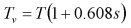The pressure at the layer top is computed from the bottom pressure and the virtual temperature, which is assumed to be constant throughout the layer:The next step is the computation of the geometrical relation between the observation point P and the air mass element Q. The surface topography was used as given in the atmospheric models. The elevation angle Gamma and the distance d between both points are obtained from the spherical distance Alpha and the height of the cell centre above ground z: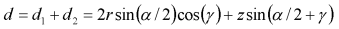with r being the radius of a spherical Earth. Using the density and the distance, the point mass attraction of a cell with volume V can be computed according to: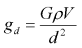where G is the gravitational constant. The three components of gravitational acceleration in a local coordinate system with x pointing towards east, y towards north and z upwards are derived from trigonometrical relationships: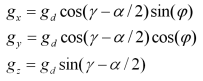where Phi is the azimuth of the mass point. All contributions are summed up component-wise.

### Local cylinder model

Since the point mass approximation is inadequate in the local zone, the spatial extent of the masses must be taken into account. For a number of cells (usually 9) around the observation point these cells are replaced by a cylinder of the same base area, which is divided into a pile of disks matching the layers of the weather model. The density within each disk is computed from the surface pressure and the virtual temperature of the corresponding layer, analogous to the regional model. The vertical attraction of each disk is computed using the analytical expression:where zbot and ztop are the heights of the disk's base and top above ground and r is the radius of the disk.

### Global 2D model

Beyond the limits of the regional model, usually 10 to 20 degrees, the contribution of the remaining atmosphere is computed assuming that the mass of the atmosphere is concentrated on the Earth's surface. The big distance justifies this simplification (see Kluegel & Wziontek 2010). Then the surface pressure p can easily expressed as a mass by:with A being the area of the cell and g the standard gravity (9.80665 m/s2). The point mass attraction reduces to: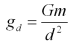### Global 3D model

Since January 2008 additional atmospheric parameters of the global model GME are available which allow the computation of the three-dimensional density distribution and thus the atmospheric attraction for each IGETS station. Following the hydrostatic equilibrium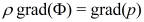the density within a layer bounded by two model levels (indices bot and top) is computed from the given pressure and the potential according to: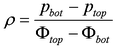The potential accounts for all masses including water vapour, cloud water, cloud ice, rain and snow. In the classical approach only water vapour is considered.

The evaluation of the geometric relation between the mass points and the observation point is identical to the regional 3D model.

### Refinement in the local zone

In order to overcome the disadvantages of the local cylinder model (topography and horizontal density gradients can't be considered) a point mass approach with refined discretization in the local zone was used for the global 3D model. Within a model cell heigths and densities are interpolated from the grid points confining the cell. The height of the observation point is projected on the model surface. The required discretization not to exceed an error threshold of 0.3 nm/s2 was evaluated in simple discretization models using an analytical solution as reference (Klügel and Wziontek 2013). Based on these results the innermost cell was subdivided 1000 times, the innermost ring of 6 cells 200 times, and each cell within an area up to a radius of 10 times the grid spacing was subdivided 20 times.